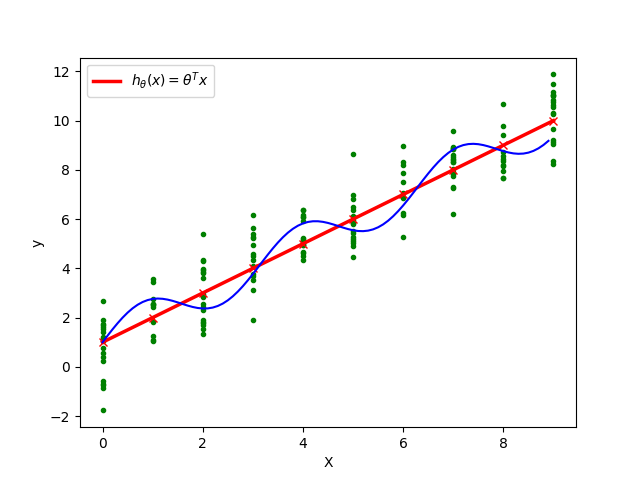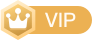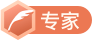# 最小二乘法的极大似然解释

最开始学习机器学习的时候，首先遇到的就是回归算法，回归算法里最最重要的就是最小二乘法，为什么损失函数要用平方和，而且还得是最小？仔细想想最小二乘法视乎很合理，但是合理在哪，怎么用数学方法来证明它合理。

J(θ)=12i=1m(hθ(x(i))y(i))2

在真实数据中，一个x值可能对应多个y值，因为实际y值可能是受多种因素影响，所以我们可以假设任意一个x对于的y的真实值服从正态分布。我们什么时候可以认为模型 hθ(x) $h_\theta(x)$ 拟合出来的点最好？当然是 hθ(x) $h_\theta(x)$ 取值概率最大的时候。如上图，红蓝两条线来拟合绿色的这些数据点，明显红色的直线拟合效果更好一些。为什么？仔细看图中直线上红色x点，红色的x点正好是当前x值下，训练数据中出现概率最高的位置（之前我们已经假设每个位置y值符合高斯分布）。所以我们要求的就是使得拟合出的线(高纬度是超平面)上概率最大的 θ $\theta$，这个时候我们就可以用到极大似然估计。
接下来我们用极大似然来证明最小二乘法。假设误差 ε(i)(1im) $ε^{(i)} (1≤i≤m)$（就是上图中绿色数据点到红色x点的距离）是独立同分布的，服从均值为0，方差为某定值 σ2 $\sigma^2$的高斯分布，我们可以得到似然函数。
y(i)=θTx(i)+ϵ(i)p(ϵ(i))=12πσe(ϵ(i))22σ2p(y(i)|x(i);θ)=12πσe((y(i)θTx(i))22σ2)

L(θ)=i=1mp(y(i)|x(i);θ)=i=1m12πσe((y(i)θTx(i))22σ2)

对上面似然函数求对数得到对数似然函数 (θ) $\ell(\theta)$
(θ)=logL(θ)=logi=1m12πσe((y(i)θTx(i))22σ2)=i=1m12πσe((y(i)θTx(i))22σ2)=mlog12πσ1σ212i=1m(hθ(x(i))y(i))2

上式中， σ $\sigma$ 是定值，我们要使得上式最大，就得使 12mi=1(hθ(x(i))y(i))2 $\frac{1}{2}\sum_{i=1}^m(h_\theta(x^{(i)}) - y^{(i)})^2$最小，于是我们就得到了最小二乘。
J(θ)=12i=1m(hθ(x(i))y(i))2

其实通过这个公式我们可以求得关于 θ $\theta$的解析解，可以直接计算出 θ $\theta$，但我们一般不这么做，因为求解析解过程中需要求矩阵的逆，这是一个非常耗时的工作（时间复杂度 Θ(n3) $\Theta(n^3)$），另外矩阵也不一定可逆，一般都是用梯度下降。但我们还是看下如何求 θ $\theta$的解析解。
J(θ)=12i=1m(hθ(x(i))y(i))2=12(Xθy)T(Xθy)

J(θ) $J(\theta)$求一阶导得到梯度。
θJ(θ)=θ(12(Xθy)T(Xθy))=θ(12(θTXTyT)(Xθy))=θ(12(θTXTXθθTXTyyTXθ+yTy))=12(2XTXθXTy(yTX)T)=XTXθXTy

XTXθXTy=0XTXθ=XTyθ=(XTX)1XTy

xindooCSDN认证博客专家 Linux 分布式 Spring

02-02433704-131万+
04-24417
05-21247
06-117906
12-295463
03-094万+
08-01328
03-23761
08-02330
03-09441
06-258475
03-072251
05-165313
01-09466
03-145842
11-156383
10-143480
©️2020 CSDN 皮肤主题: 博客之星2020 设计师:CY__